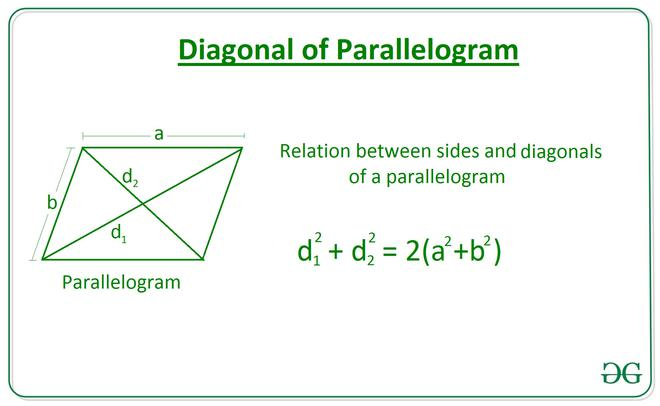# Length of a Diagonal of a Parallelogram using the length of Sides and the other Diagonal

• Last Updated : 17 Mar, 2021

Given two integers A and B, denoting the length of a parallelogram and an integer D, denoting the length of a diagonal, the task is to find the length of another diagonal of the parallelogram.

Examples:

Input: A = 10, B = 30, D = 20
Output: 40.0

Input: A = 6, B = 8, D = 10
Output: 10.0

Approach:
The relation between sides and diagonals of a parallelogram length of diagonal is given by the equation:Below is the implementation of the above approach:

## C++

 // C++ Program to implement // the above approach #include  using namespace std;   // Function to calculate the length // of the diagonal of a parallelogram // using two sides and other diagonal float Length_Diagonal(int a, int b, int d) {       float diagonal = sqrt(2 * ((a * a) +                                 (b * b)) - (d * d));       return diagonal; }   // Driver Code int main() {     int A = 10;     int B = 30;     int D = 20;       // Function Call     float ans = Length_Diagonal(A, B, D);       // Print the final answer     printf("%0.1f", ans);     return 0; }   // This code is contributed by Rohit_ranjan

## Java

 // Java Program to implement // the above approach class GFG{   // Function to calculate the length // of the diagonal of a parallelogram // using two sides and other diagonal static float Length_Diagonal(int a, int b, int d) {       float diagonal = (float) Math.sqrt(2 * ((a * a) +                                   (b * b)) - (d * d));       return diagonal; }   // Driver Code public static void main(String[] args) {     int A = 10;     int B = 30;     int D = 20;       // Function Call     float ans = Length_Diagonal(A, B, D);       // Print the final answer     System.out.printf("%.1f", ans); } }   // This code is contributed by Rajput-Ji

## Python

 # Python Program to implement # the above approach   import math   # Function to calculate the length # of the diagonal of a parallelogram # using two sides and other diagonal def Length_Diagonal(a, b, d):       diagonal = math.sqrt(2 * ((a**2) \     + (b**2)) - (d**2))       return diagonal     # Driver Code A = 10 B = 30 D = 20   # Function Call ans = Length_Diagonal(A, B, D)   # Print the final answer print(round(ans, 2))

## C#

 // C# Program to implement // the above approach using System; class GFG{   // Function to calculate the length // of the diagonal of a parallelogram // using two sides and other diagonal static float Length_Diagonal(int a, int b, int d) {       float diagonal = (float) Math.Sqrt(2 * ((a * a) +                                   (b * b)) - (d * d));       return diagonal; }   // Driver Code public static void Main(String[] args) {     int A = 10;     int B = 30;     int D = 20;       // Function Call     float ans = Length_Diagonal(A, B, D);       // Print the readonly answer     Console.Write("{0:F1}", ans); } }   // This code is contributed by Rajput-Ji

## Javascript

 

Output:

40.0

Time Complexity: O(1)
Auxiliary Space: O(1)

My Personal Notes arrow_drop_up
Recommended Articles
Page :